Algebraic General Topology. Vol 1: Paperback / E-book || Axiomatic Theory of Formulas: Paperback / E-book

As an Amazon Associate I earn from qualifying purchases.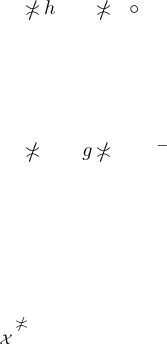Multidimensional Funcoids
by Victor Porton
78640, Shay Agnon 32-29, Ashkelon, Israel
Web: http://www.mathematics21.org
Abstract
First I deﬁne a product of two funcoids. Then I dene multifuncoids and staroids as gen-
eralizations of funcoids. Using staroids I deﬁne a product of an arbitrary (possibly inﬁnite)
family of funcoids and some other products.
1 Draft status
It is a rough draft.
2 Notation
This article presents a ge ne ralization of concepts from  and .
In this article I will use to denote order in a poset and , to denote meets and joins on a
semilattice. I reserve , , and for set-theoretic supset-relation, intersection, and union.
For a poset A I will denote Least(A) the set of least elements of A. (This set always has eithe r
one or z ero elements.)
With this notation we do not need the conc ept of ﬁlter objects (), we will use the standard
set of ﬁlters, but the order on the lattice of lters will b e opposite the set theoretic inclusion
of ﬁlters.
3 Product of two func oids
3.1 Lemmas
Lemma 1. Let A, B, C are sets, f FCD(A; B), g FCD(B; C), h FCD(A; C). Then
g f
h g
h f
1
.
Proof. See .
Lemma 2. Let A, B, C are sets, f RLD(A; B), g RLD(B; C), h RLD(A; C). Then
g f
h g
h f
1
.
Proof. See .
Lemma 3. f (A ×
FCD
B) = A ×
FCD
hf iB for elements A A a nd B B of some posets A, B with
least elements and f FCD(A; B).
Proof. hf (A ×
FCD
B)iX =
hf iB if X
A
0 if X A
= hA ×
FCD
hf iBiX .
1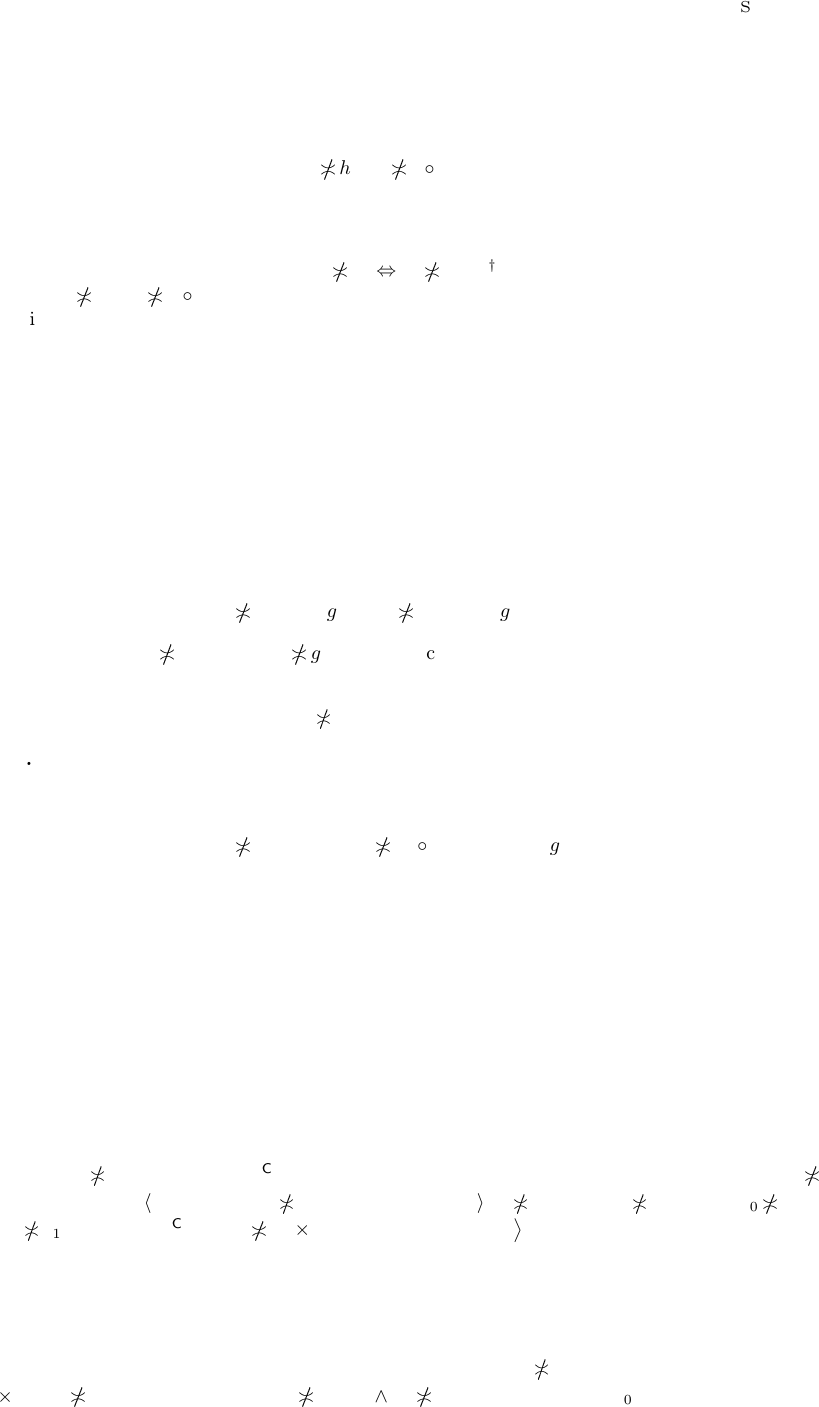3.2 Deﬁnition
Deﬁnition 4. I will call a quasi-invertible category a partially ordered dagger catego ry such that
it holds
g f
h g
h f
(1)
for every morphisms f Hom(A; B), g Ho m(B; C), h Hom(A; C), where A, B, C are objects
of this category.
Inverting this formula, we get f
g
h
g
f h
. After replacement of variab le s, this
gives: f
g
h g
f h
As it follows from , the cate gory of funcoids and the category of reloids are quasi-invertible
(taking f
= f
1
). Moreover by  the category of pointfree funcoids between lattices of ﬁlters on
boolean lattices are quasi-invertible.
Deﬁnition 5. The cross-composition product of morphisms f and g of a quasi-invertible category
is the pointfree funcoid Ho m(Src f; Src g) Hom(Dst f ; Dst g) deﬁned by the formulas (for every
a Hom(Src f ; Src g) and b Hom(Dst f ; Dst g)):
f ×
(C)
g
a = g a f
and

f ×
(C)
g
1
b = g
b f .
The cross-composition product is a po intfree funcoid from Hom(Src f ; Src g) to Hom(Dst f ; Dst g).
We need to prove that it is really a pointfree funcoid that is that
b
f ×
(C)
g
a a

f ×
(C)
g
1
b.
This formula means b
g a f
a
g
b f and can be ea sily proved applying the formula (1)
two times.
Proposition 6. a
f ×
(C)
g
b a f
g
b.
Proof. From the lemma.
Proposition 7. a
f ×
(C)
g
b f
a ×
(C)
b
g.
Proof. f
a ×
(C)
b
g f a
b
g a f
g
b a
f ×
(C)
g
b.
Theorem 8.
f ×
(C)
g
= f
×
(C)
g
.
Proof. For every funcoids a Hom(Src f ; Src g) and b Hom(Dst f; D st g) we have:

f ×
(C)
g
b = g
b f = g
b f =
f
×
(C)
g
b.

f ×
(C)
g
a =
f ×
(C)
g
a = g a f
=

f
×
(C)
g
a.
Theorem 9. Let f , g are morphisms of a quasi-invertible ca tegory where Dst f and Dst g are f.o.
on boolean lattices. Then for every f.o. A
0
F(Src f), B
0
F(Src g)
f ×
(C)
g
(A
0
×
FCD
B
0
) = hf iA
0
×
FCD
hgiB
0
.
Proof. For every atom a
1
×
FCD
b
1
(a
1
atoms
Dst f
, b
1
atoms
Dst g
) of the latt ice of funcoids we have:
a
1
×
FCD
b
1
f ×
(C)
g
(A
0
×
FCD
B
0
) A
0
×
FCD
B
0
f ×
(C)
g
a
1
×
FCD
b
1
(A
0
×
FCD
B
0
) f
g
(a
1
×
FCD
b
1
) hf iA
0
×
FCD
B
0
a
1
×
FCD
hg
ib
1
hf iA
0
a
1
hg
ib
1
B
0
hf iA
0
a
1
hgiB
0
b
1
hf iA
0
×
FCD
hgiB
0
a
1
×
FCD
b
1
. Thus
f ×
(C)
g
(A
0
×
FCD
B
0
) = hf iA
0
×
FCD
hgiB
0
because the lattice FCD(F(Dst f); F(Dst g)) is atomically separable (corollary 64 in ).
Proposition 10. A
0
×
FCD
B
0
f ×
(C)
g
A
1
×
FCD
B
1
A
0
[f] A
1
B
0
[g] B
1
for every A
0
F(Src f ),
A
1
F(Dst f ), B
0
F(Src g), B
1
F(Dst g).
Proof. A
0
×
FCD
B
0
f ×
(C)
g
A
1
×
FCD
B
1
A
1
×
FCD
B
1
f ×
(C)
g
(A
0
×
FCD
B
0
)
A
1
×
FCD
B
1
hf iA
0
×
FCD
hgiB
0
A
1
hf iA
0
B
1
hgiB
0
A
0
[f ] A
1
B
0
[g] B
1
.
2 Section 3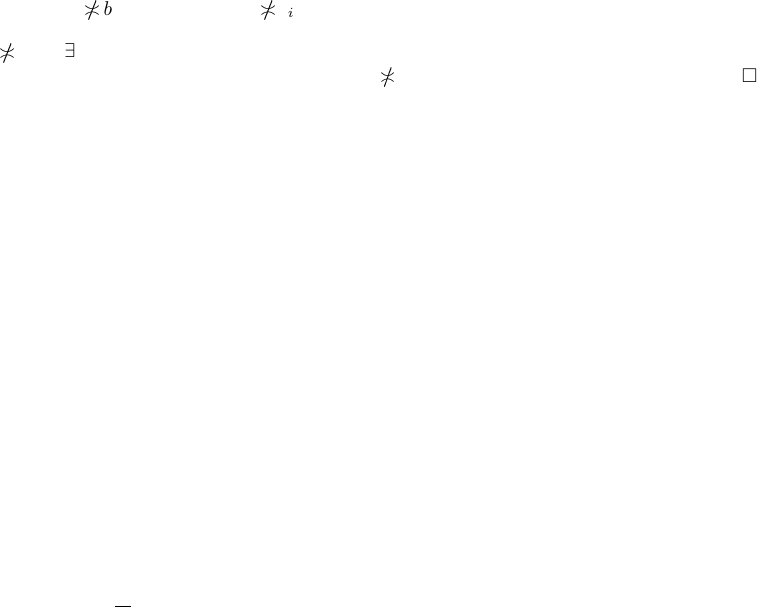4 Function spaces of posets
Deﬁnition 11. Let A
i
is a family of pose ts indexed by some set dom A. We will deﬁne order of
families of posets by the formula
a b i dom A: a
i
b
i
.
I will call this new poset A =
Q
A the function space of posets and the above order product order .
Proposition 12. The function space for posets is also a poset.
Proof.
Reﬂexivity. Obvi ous.
Antisymmetry. Obvious.
Transitivity. Obvious.
Obvious 13. A has least element i each A
i
has a least element. In this case
Least(A) =
Y
idom A
Least(A
i
).
Proposition 14. a
b i dom A: a
i
b
i
for every a, b
Q
A.
Proof. a
b c
Q
A: (c a c b) c
Q
Ai d om A: (c
i
a
i
c
i
b
i
)
i dom Ax
Q
A: (x a
i
x b
i
) i dom A: a
i
b
i
.
Proposition 15.
1. If A
i
are jo in-semilattices then A is a join-semilattice and
A B = λi dom A: Ai Bi. (2)
2. If A
i
are meet-semilattices then A is a meet-semilattice and
A B = λi dom A: Ai Bi. (3)
Proof. It is enough to pr ove the formula (2 ).
It’s obvious tha t λi dom A: Ai Bi A, B.
Let C A, B. Then (for every i dom A) Ci Ai and Ci Bi. Thus Ci Ai Bi that is
C λi dom A: Ai Bi.
Corollary 16. If A
i
are lattices then A is a lattice.
Obvious 17. If A
i
are distributive lattices then A is a distributive lattice.
Obvious 18. If A
i
are (co-)brouwerian lattices then A is a (co-)brouwer ian lat tice.
Proposition 19. If A
i
are boolea n lattices then
Q
A is a boolean lattice.
Proof. We need to prove only that every element a
Q
A has a complement. But this complement
is evidently λi dom a: a
i
.
Proposition 20. If A
i
are lattices then for every S P
Q
A
1.
F
S = λi dom A:
F
{x
i
| x S } wh enever
F
{x
i
| x S } exists;
2.
d
S = λi dom A:
d
{x
i
| x S } wh enever
d
{x
i
| x S } exists.
Proof. It’s enough to prove the ﬁrst formula.
(λi dom A:
F
{x
i
| x S })
i
=
F
{x
i
| x S } x
i
for every x S and i dom A.
Let y x for every x S. Then y
i
x
i
for every i dom A and thus y
i
F
{x
i
| x S} =
(λi dom A:
F
{x
i
| x S })
i
that is y λi dom A:
F
{x
i
| x S }.
Thus
F
S = λi dom A:
F
{x
i
| x S } by the deﬁnition of join.
Function spaces of posets 3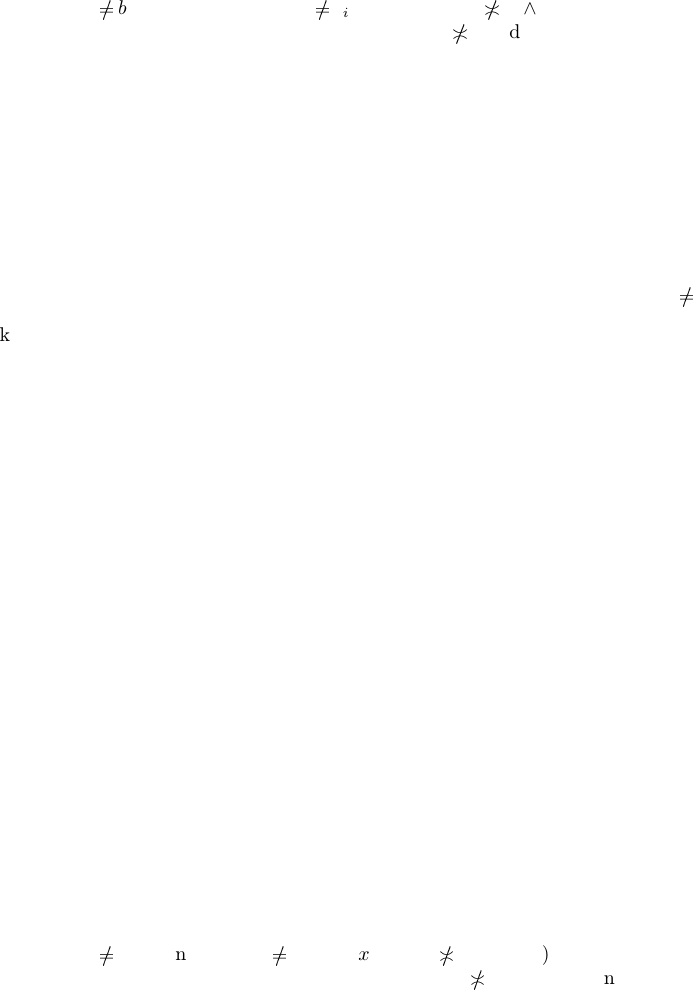Corollary 21. If A
i
are complete lattices then A is a complete lat tice.
Proposition 22. If each A
i
is a separable poset with least element (for some index set n) then
Q
A is a sepa rable poset.
Proof. Let a
b. Then i dom A: a
i
b
i
. So x A
i
: (x
a
i
x b
i
) (or vic e versa).
Take y = (((dom A) \ {i}) × {0}) {(i; x)}. Then y
a and y b.
Obvious 23. If every A
i
is a poset with least element 0
i
, then the set of atoms of
Q
A is
{({k} × atoms
A
k
) (λi (d om A) \ {k}: 0
i
) | k dom A}.
Proposition 24. If every A
i
is an atomistic poset with least element 0
i
, then
Q
A is an atomistic
poset.
Proof. x
i
=
F
atoms x
i
for every x
i
A
i
. Thus
x = λi dom x: x
i
=
G
idom x
atoms x
i
=
G
idom x
λj dom x:
x
i
if j = i
0
i
if j
i.
Take join two times.
Corollary 25. If A
i
are atomistic complete lattices, then
Q
A is atomically separable.
Proof. Proposition 14 in .
Proposition 26. L e t (A
in
; Z
in
) is a family of ﬁltrators. Then (
Q
A;
Q
Z) is a ﬁltra tor.
Proof. We need to prove that
Q
Z is a sub-poset of
Q
A. First
Q
Z
Q
A because Z
i
A
i
for each i n.
Let A, B
Q
Z and A
Q
Z
B. Then i n: A
i
Z
i
B
i
; consequently i n: A
i
A
i
B
i
that is
A
Q
A
B.
Proposition 27. L e t (A
in
; Z
in
) is a family of ﬁltrators.
1. Th e ﬁltrator (
Q
A;
Q
Z) is (ﬁnitely) j oin-closed if every (A
i
; Z
i
) is (ﬁ nitely) join- closed.
2. Th e ltrator (
Q
A;
Q
Z) is (ﬁnitely) meet-closed if every (A
i
; Z
i
) is (ﬁnitely) meet-closed.
Proof. Let every (A
i
; Z
i
) is ﬁnitely join-closed. Let A, B
Q
Z. Then A
Q
Z
B = λ n:
A
i
Z
i
B
i
= λ n: A
i
A
i
B
i
= A
Q
A
B.
Let now every (A
i
; Z
i
) is ﬁnitely jo in-closed. Let S P
Q
Z. Then
F
Q
Z
S = λi dom A:
F
Z
i
{x
i
| x S } = λi dom A:
F
A
i
{x
i
| x S } =
F
Q
A
S.
The rest follows fr om symmetry.
Proposition 28 . If each (A
i
; Z
i
) where i n (for some index set n) is a down-aligned ﬁltrator with
separable core (for some index set n) then (
Q
A;
Q
Z) is with separable core.
Proof. Let a
b. Then i n: a
i
b
i
. So x Z
i
: (x
a
i
x b
i
) (or vice versa).
Take y = ((n \ {i}) × {0}) {(i; x)}. Then we have y
a and y b and y Z.
Proposition 29. Let every A
i
is a bounded lattice. Every (A
i
; Z
i
) is a ce ntral ltrator iﬀ (
Q
A;
Q
Z) is a central ﬁltrator.
Proof. x Z(
Q
A) y
Q
A:
x y = 0
Q
A
x y = 1
Q
A
y
Q
Ai dom A:
(x
i
y
i
= 0
A
i
x
i
y
i
= 1
A
i
) i dom Ay A
i
: (x
i
y
i
= 0
A
i
x
i
y
i
= 1
A
i
) i dom A:
x
i
Z(A
i
).
Proposition 30. For e very element a of a product ﬁltrator (
Q
A;
Q
Z):
1. up a =
Q
idom a
up a
i
;
4 Section 4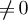2. down a =
Q
idom a
down a
i
.
Proof. We will prove only t he ﬁr st as the second is dual.
up a = {c
Q
Z | c a} = {c
Q
Z | i dom a: c
i
a
i
} = {c
Q
Z | i dom a:
c
i
up a
i
} =
Q
idom a
up a
i
.
Proposition 31. If every (A
i
; Z
i
) is a ﬁltered complete lattice ﬁltrator, then (
Q
A;
Q
Z) is a
ﬁltered complete lattice ﬁltrator.
Proof. That
Q
A is a complete lattice is already proved above. We have for every a
Q
A
d
Q
A
up a = λi dom A:
d
{x
i
| x up a} = λi dom A:
d
{x | x up a
i
} = λi dom A:
d
up a
i
= λi dom A: a
i
= a.
Obvious 32. If every (A
i
; Z
i
) is a preﬁltered co mplete lattice ﬁltrator, then (
Q
A;
Q
Z) is a
preﬁltered complete lattice ﬁlt rato r.
Proposition 33. Let A
i
is a non -empty poset. Every (A
i
; Z
i
) is a semiﬁltered complete lattice
ﬁltrator iﬀ (
Q
A;
Q
Z) is a semiﬁltered complete lattice ﬁltrator.
Proof. up a up b λi dom A: up a
i
up b
i
λi dom A: a
i
b
i
a b for e very a, b
Q
A
(used the fact that up a
i
0 because up is injective).
Proposition 34. L e t (A
i
; Z
i
) a re ﬁl trators and each Z
i
is a complete lattice. Fo r a
Q
A:
1. Cor a = λi dom a: Cor a
i
;
2. Cor
a = λi dom a: Cor
a
i
.
Proof. We will prove only t he ﬁr st, because the second is dual.
Cor a =
d
Q
Z
upa = λi dom a:
d
Z
i
{x
i
| x up a} = λi doma:
d
Z
i
{x | x upa
i
}= λi doma:
d
Z
i
up a
i
= λi dom a: Cor a
i
.
Proposition 35. If each (A
i
; Z
i
) is a ﬁltrator with (co-)separable core, then (
Q
A;
Q
Z) is a
ﬁltrator with (co-)separable core.
Proof. We will prove only f or separable core, as co-separable core is dual.
x
Q
A
y i dom A: x
i
A
i
y
i
i dom AX up x
i
: X
A
i
y
i
X up xi dom A:
X
i
A
i
y
i
X up x: X
Q
A
y for every x, y
Q
A.
Obvious 36.
1. If each (A
i
; Z
i
) is a down-aligned ltrator, then (
Q
A;
Q
Z) is a down-aligned ltrator.
2. If each (A
i
; Z
i
) is an up-aligned ﬁltrator, then (
Q
A;
Q
Z) is an up-aligned ﬁltrator.
Proposition 37. If every b
i
is substractive from a
i
where a and b are n-indexed families of
distributive lattices with le ast elements (where n is an index set), then a \ b = λi n: a
i
\ b
i
.
Proof. We need to prove (λi n: a
i
\ b
i
) b = 0 and a b = b (λi n: a
i
\ b
i
).
Really, (λi n: a
i
\ b
i
) b = λi n: (a
i
\ b
i
) b
i
= 0 and b (λi n: a
i
\ b
i
) = λ i n:
b
i
(a
i
\ b
i
) = λi n: b
i
a
i
= a b.
Proposition 38. If every A
i
is a distributive lattice, then a \
b = λ i dom A: a
i
\
b
i
for every a,
b
Q
A whenever every a
i
\
b
i
is de ﬁned.
Proof. We need to prove that λi dom A: a
i
\
b
i
=
d
{z
Q
A | a b z }.
To prove it is enough to show a
i
\
b
i
=
d
{z
i
| z
Q
A, a b z} that is a
i
\
b
i
=
d
{z A
i
| a
i
b
i
z} what is true by deﬁnition.
Proposition 39. If every A
i
is a dis tributive lattice with least element, then a#b = λi dom A:
a
i
#b
i
for every a, b
Q
A whenever every a
i
#b
i
is de ﬁned.
Function spaces of posets 5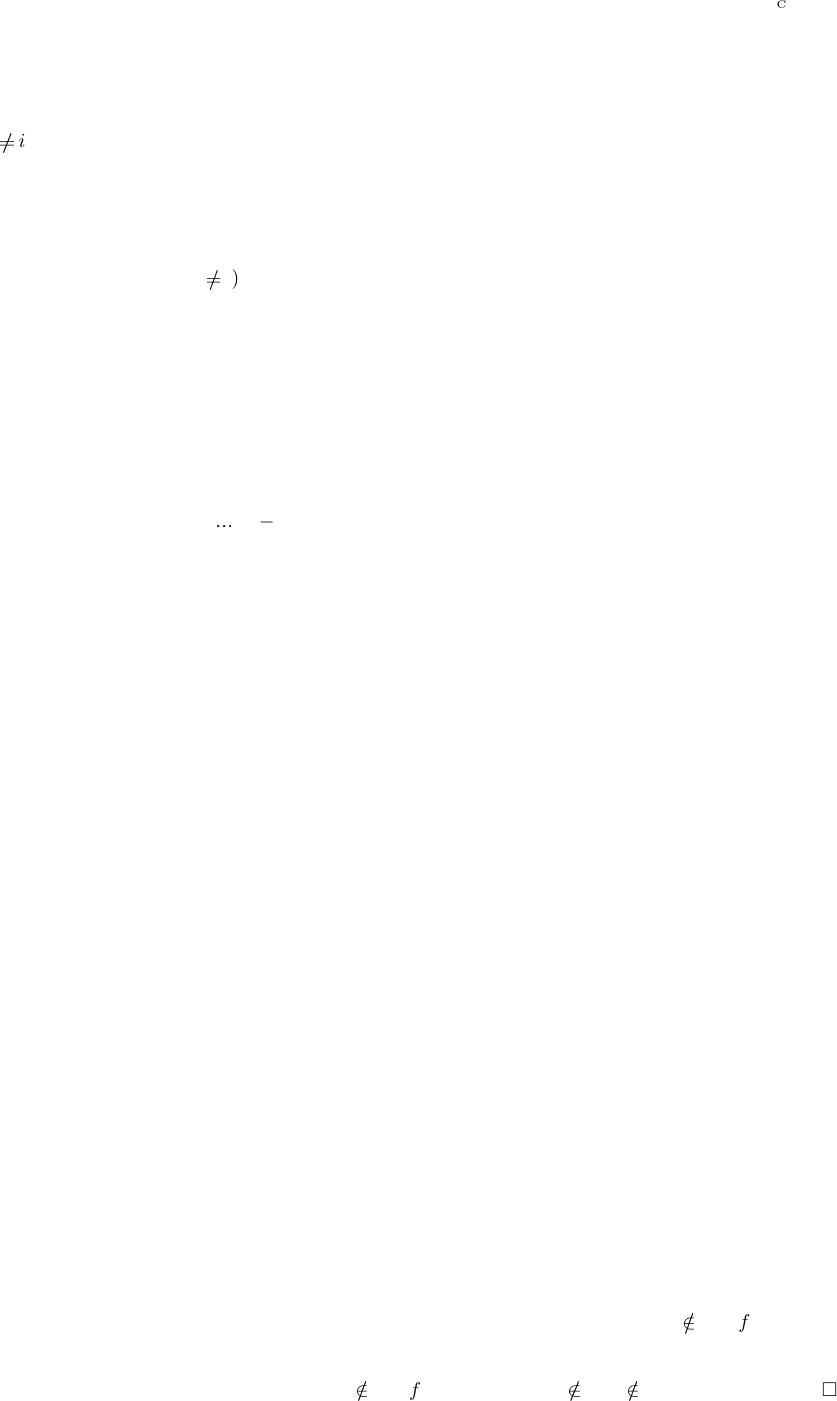Proof. We need to prove that λi dom A: a
i
#b
i
=
d
{z
Q
A | z a z b}.
To prove it is enough to show a
i
#b
i
=
d
{z
i
| z
Q
A, z a z b} that is a
i
#b
i
=
d
{z A
i
| z a
i
j dom A: z
j
b
j
} that is a
i
#b
i
=
d
{z A
i
| z a
i
z b
i
} (take z
i
= 0 for
j
i) what is true by deﬁnition.
Proposition 40. Let every A
i
is a poset with least element and a
i
is deﬁned. Then a
= λi n: a
i
.
Proof. We need to prove that λi dom A: a
i
=
F
{c A | c a}. To prove this it is enough to show
that a
i
=
F
{c
i
| c A, c a} th at is a
i
=
F
{c
i
| c A, j n: c
j
a
j
} that is a
i
=
F
{c
i
| c A,
c
i
a
i
} (take c
i
= 0 for j
i) tha t is a
i
=
F
{c A | c a
i
} what is true by deﬁnition.
Corollary 41. Let every A
i
is a poset with le ast element and a
i
+
is deﬁned. Then a
+
= λi n: a
i
+
.
Proof. By duality.
5 Deﬁnition o f staroids
Let n be a set. As an example, n may be an ordinal, n may be a natural number, considered as a
set by the fo rmula n = {0,
, n 1}. Let A = A
in
is a family of posets indexed by the set n.
Deﬁnition 42. I will call an anchored relation a pair f = (form f ; GR f) of a family form(f ) o f
sets in de xed by the some index set and a relation GR(f ) P
Q
form(f ). I call GR(f) the graph
of the anchored relation f. I denote Anch(A) the set of small anchored relations of the form A.
Deﬁnition 43. An anchored relation on powersets is an anchored relation f such that every
(form f )
i
is a powerset.
I will denote arity f = dom form f .
Deﬁnition 44 . Every set of anchored relations of the same form constitutes a poset by the formula
f g GR f GR g.
Deﬁnition 45. An anchored relation is an anchored relation between posets when every (form f )
i
is a poset .
Deﬁnition 46. Let f is an anchored relation. For every i arity f and L
Q
((form f)|
(arity f)\{i}
)
(val f )
i
L = {X (form f )
i
| L {(i; X)} GR f }
(“val” is an abbreviation of the word “value”.)
Obvious 47. X (val f )
i
L L {(i; X)} GR f .
Proposition 48. f can be restored knowing form(f ) and (val f)
i
for some i n.
Proof. GR f = {K
Q
form f | K GR f } = {L {(i; X)} | L
Q
(form f)|
(arity f)\{i}
,
X (form f)
i
, L {(i; X)} GR f } = {L {(i; X)} | L
Q
(form f)|
(arity f)\{i}
, X (val f )
i
L}.
Deﬁnition 49. A pre-staroid is an anchored relation f between poset such that (val f )
i
L is a free
star for every i arity f , L
Q
(form f )|
(arity f)\{i}
.
Deﬁnition 50. A staroid is a pre-staroid whose graph is an upper set (on the poset if anchored
relations of the form of this pre-staroid).
Proposition 51. If L
Q
form f and L
i
= 0
(form f)
i
for some i arity f t he n L
f if f is an pre-
staroid.
Proof. Let K = L|
(arity f)\{i}
. We have 0
(val f )
i
K; K {(i; 0)}
f; L
f.
6 Section 5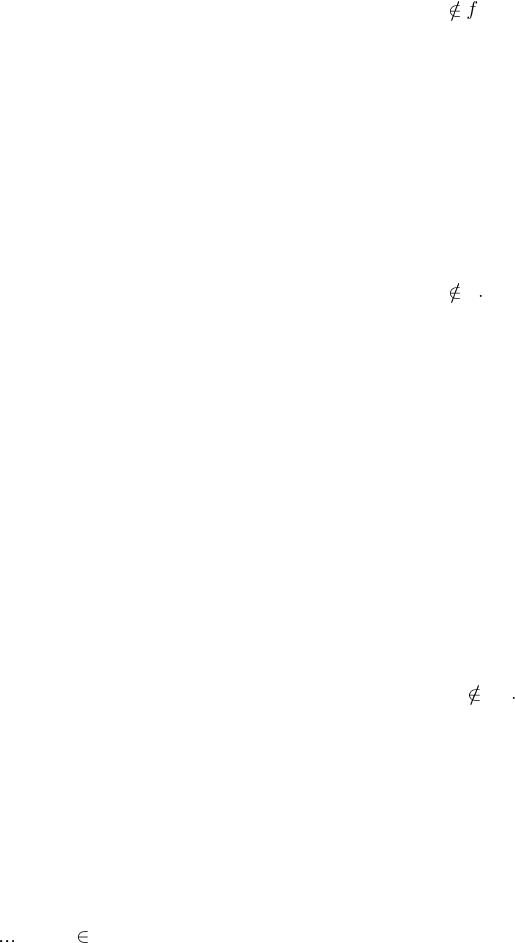Deﬁnition 52. Inﬁnitary pre-staroid is such a staroid whose arity is inﬁnite; ﬁnitary pre-staroid
is such a staroid whos e arity is ﬁnite.
Next we will deﬁne com pletary staroids. First goes the general case, next simpler case for the
special case of join-semilattices instead of arb itr ary posets.
Deﬁnition 53. A completary staroid is a poset relation conforming to the formulas:
1. K
Q
form f: (K L
0
K L
1
K GR f ) c {0, 1}
n
: (λi n: L
c(i)
i) GR f for
every L
0
, L
1
Q
form f.
2. If L
Q
form f and L
i
= 0
(form f)
i
for some i arity f then L
f.
Lemma 54. Every completary staroid is an upper set.
Proof. Let f is a completary staroid. Let L
0
L
1
for some L
0
, L
1
Q
form f and L
0
f.
Then taking c = n × {0} we get λi n: L
c(i)
i = λ i n: L
0
i = L
0
f and thus L
1
f because
L
1
L
0
L
1
L
1
.
Proposition 55. A relation between posets whose form is a family of join-semilattices is a com-
pletary staroid iﬀ both:
1. L
0
L
1
GR f c {0, 1}
n
: (λi n: L
c(i)
i) GR f for every L
0
, L
1
Q
form f .
2. If L
Q
form f and L
i
= 0
(form f)
i
for some i arity f then L
f.
Proof. Let the formulas (1) and (2) hold. Then f is an upper set: Let L
0
L
1
for some L
0
,
L
1
Q
form f a nd L
0
f . Then taking c = n × {0} we get λi n: L
c(i)
i = λi n: L
0
i = L
0
f and
thus L
1
= L
0
L
1
f .
Thus to ﬁnish the proof it is enough to show that
L
0
L
1
GR f K
Y
form f : (K L
0
K L
1
K GR f )
under condition that GR f is an upper set. But this is obvious.
Proposition 56. A completary s taroid is a staroid.
Proof. Let f is a co mpletary s taroid.
Let K
Q
i(arity f)\{i}
(form f)
i
. Let L
0
= K {(i; X
0
)}, L
1
= K {(i; X
1
)} for some X
0
,
X
1
A
i
. Then X
0
X
1
(val f )
i
K L
0
L
1
GR f k {0, 1}: K {(i; X
k
)} GR f K {(i;
X
0
)} f K {(i ; X
1
)} GR f X
0
(val f)
i
K X
1
(val f)
i
K.
So (val f )
i
K is a free star (taken in account that K
i
= 0
(form f)
i
f
K).
f is an upper set by the lemma.
Lemma 57. Every ﬁnitary pre -staroid is completary.
Proof. c {0, 1 }
n
: (λi n: L
c(i)
i) GR f c {0, 1}
n1
: ({(n 1; L
0
(n 1))} (λi n 1:
L
c(i)
i)) GR f ({(n 1; L
1
(n 1))} (λ i n 1: L
c(i)
i)) GR f c {0, 1}
n1
:
L
0
(n 1) (val f )
n1
(λi n 1: L
c(i)
i) L
1
(n 1) (val f )
n1
(λi n 1: L
c(i)
i) c { 0,
1}
n1
K
Q
form f:(K L
0
(n 1) K L
1
(n 1) K (val f)
n1
(λi n 1:L
c(i)
i)) c {0,
1}
n1
K
n1
(form f )
n1
: (K
n1
L
0
(n 1) K
n1
L
1
(n 1) {(n 1; K)} (λi n 1:
L
c(i)
i)) GR f
K
Q
form f : (K L
0
K L
1
K GR f ).
Exercise 1. Prove the simpler special case of the above theorem when the form is a family of join-semilattices.
Theorem 58. For ﬁnite arity the following are the same:
1. pre-staroids;
2. star oids;
Definition of staroids 7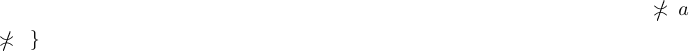3. completary sta roids.
Proof. f is a ﬁnitary pre- staroid f is a ﬁnitary comple tary staroid.
f is a ﬁnitary completary s taroid f is a ﬁnitary staroid.
f is a ﬁnitary staroid f is a nitary pre-staroid.
Deﬁnition 59. We will denote the set of staroids, pre-staroids, and completary staroids o f a form
A correspondingly as Strd(A), pStrd(A), and cStrd(A).
Let x a ﬁltrator (A; Z).
Deﬁnition 60. f = f Z for every f PA (downgrading f ).
Deﬁnition 61. f = {L A | up L f } for every f PZ (upgrading f ).
Obvious 62. a f up a f for every f PZ and a A.
Proposition 63. f = f if f is an upper set.
Proof. f = f Z = {L Z | up L f } = {L Z | up L f } = f PZ = f .
Let x a family (A; Z) of ﬁltrators.
For a graph f o f a staroid deﬁne f and f taking the ﬁltrator of (
Q
A;
Q
Z).
For a staroid f deﬁne:
form f = Z and GR f = GR f ;
form f = A and GR f = GR f .
Proposition 64. (val f ))
i
L = (val f)
i
L Z
i
for eve ry L
Q
Z|
(arity f)\{i}
.
Proof. (val f ))
i
L = {X (form f )
i
| L {(i; X)} GR f
Q
Z} = {X Z
i
| L {(i;
X)} GR f } = (val f )
i
L Z
i
.
Proposition 65. Let (A
i
; Z
i
) are ﬁnitely join -closed ﬁltrators with both the base and the core
being join-semilat tices. If f is a staroid of the form A, then f is a staroid of the form Z.
Proof. Let f is a a staroid.
We need to prove that (val f )
i
L is a free star. It follows from the last proposition and the
fact that it is join-closed.
Proposition 66.
Q
Strd
a = ⇈
Q
Strd
a if each a
i
A
i
(for i n where n is some index set) where
A
i
is a separable poset with least element.
Proof. ⇈
Q
Strd
a =
L
Q
A | L
Q
Strd
a
= {L
Q
A | K L: K
a} =
{L
Q
A | L
a} =
Q
Strd
a (taken into acco unt that
Q
A is a sepa rable poset).
6.2 Displacement
Deﬁnition 67. Let f is an indexed family of po intfree funcoids. The displacement of the pre-
staroid
p A = pStrd(λi dom f : FCD(Src f
i
; Src g
i
))
8 Section 6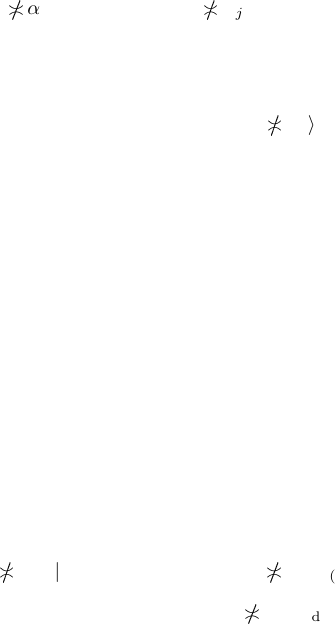is de ned as a staroid
q B = pStrd(λi dom f: RLD(Src f
i
; Src g
i
))
such that
q =
(B;C;
B
)
(A;C;
A
)
p
where C = pStrd
Q
idom f
Src f
i
;
Q
idom f
Dst f
i
.
Deﬁnition 68. We w ill deﬁne displaced product of a family f of funcoids by the formula:
Q
(DP)
f = DP
Q
(C)
f
.
Remark 69. The interesting aspect of displaced product of funcoids is that displaced product of
pointfree funcoids is a funcoid (not just a pointfree funcoid).
7 Multifun coids
Deﬁnition 70. I call an pre-multifuncoid sketch f of the form A (where every A
i
is a poset) the
pair (A; α) where for every i dom α
α
i
:
Y
A|
(dom A)\{i}
A
i
.
I denote hf i = α.
Deﬁnition 71. A pre-multifuncoid sketch on powersets is a pre-multifuncoid sketch such that
every A
i
is the set of ﬁlters on a powerset.
Deﬁnition 72. I will call a pre-multifuncoi d a pre-multifuncoid sketch s uch that for every i,
j dom A and L
Q
A
L
i
α
i
L|
(dom L)\{i}
L
j
α
j
L|
(dom L)\{j }
. (4)
Deﬁnition 73. Let A is an indexed fa mily of starrish posets. The pre-staroid correspondi ng to a
pre-multifuncoid f is [f] deﬁned by the formula:
form [f ]=A and L GR [f]L
i
hf i
i
L|
(dom L)\{i}
.
Proposition 74. The pre-staroid corresponding to a pre-multifuncoid is really a pre-staroid.
Proof. By the deﬁnition of starrish posets.
Deﬁnition 75. I will call a multifuncoid a pre-multifuncoid to which corresponds a staroid.
Deﬁnition 76. I will call a completary multifuncoid a pre-multifuncoid to which corresponds a
completary staroid .
Theorem 77. Fix some indexed family A of bo olean lattices. The the set of multifuncoids g
bijectively corresponds to set of pre-staroids f of form A by the formulas:
1. f = [g] for every i dom A, L
Q
A;
2. hgi
i
L = (val f )
i
L.
Proof. Let f is a pre-staroid of the form A. If α is deﬁned by the formula α
i
L = hf i
i
L then
∂α
i
L = (val f )
i
L. Then
L
i
α
i
L|
(dom L)\{i}
L f L
j
α
j
L|
(dom L)\{j }
.
For the sta roid f
deﬁned by the formula L f
L
i
α
i
L|
(dom L)\{i}
we have:
L f
L
i
∂α
i
L|
(dom L)\{i}
L
i
(val f)
i
L|
(dom L)\{i}
L f ;
Multifuncoids 9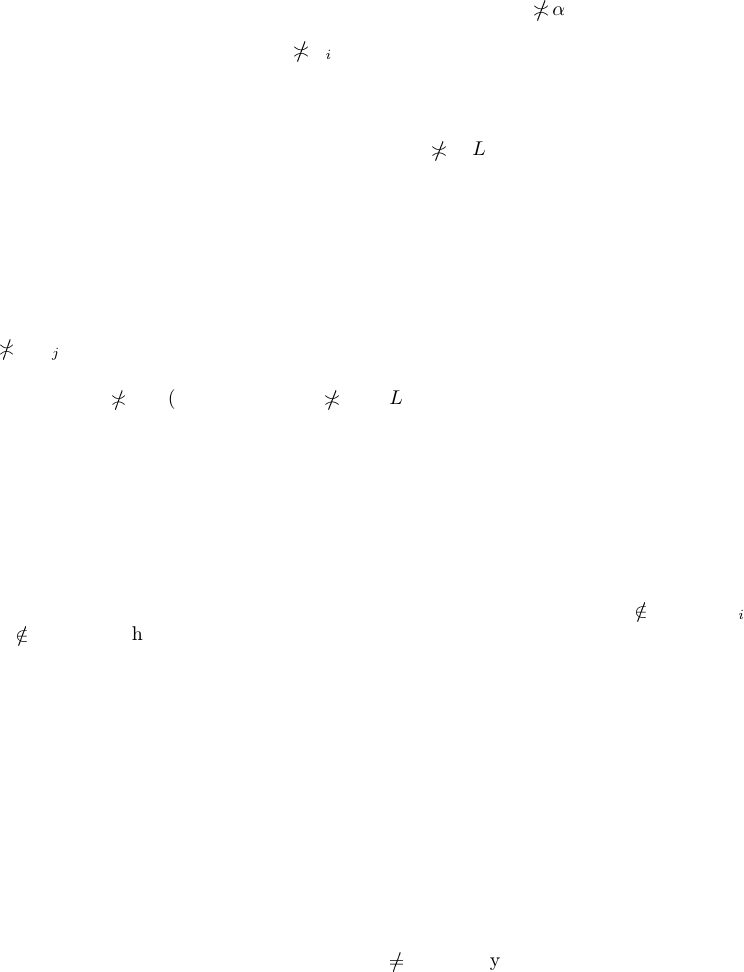thus f
= f.
Let now α is an indexed fa mily of functions α
i
A
i
(dom A)\{i}
conforming to the formula (4).
Let relation f b e tween posets is deﬁned by the formula L f L
i
α
i
L|
(dom L)\{i}
. T he n
(val f )
i
L = {K A
i
| K
α
i
L|
(dom L)\{i}
} = K = ∂α
i
L|
(dom L)\{i}
and thus (val f)
i
L is a c ore star that is f is a pre-staroid. For the indexed family α
deﬁned by
the formula α
i
L = hf i
i
L we have
∂α
i
L = hf i
i
L = {K A
i
| K
α
i
L} = ∂α
i
L;
thus α
= α.
We have shown that these are bijections.
Theorem 78. hf i
j
(L {(i; X Y )}) = hf i
j
(L {(i; X)}) hf i
j
(L {(i; Y )}) for every staroid f
if (form f )
j
is a boolean lattice and i, j arity f.
Proof. Let i arity f and L
Q
kL \{i,j }
A
k
. Let Z A
i
.
Z
hf i
j
(L {(i; X Y )}) L {(i; X Y ), (j; Z)} f X Y (va l f )
i
(L {(j;
Z)}) X (val f)
i
(L {(j; Z)} Y (va l f)
i
(L {(j; Z)}) L {(i; X), (j; Z)} f L {(i; Y ),
(j; Z)} f
A
i
Z
hf i
j
(L {(i; X)}) Z
hf i
j
(L {(i; Y )})
Thus hf i
j
(L {(i; X Y )}) = h f i
j
(L {(i; X)}) hf i
j
(L {(i; Y )}).
Let us cons ider the ﬁltrator
Q
iarity f
F((form f )
i
);
Q
iarity f
(form f )
i
.
Theorem 79. Let (A
i
; Z
i
) is a family of join-closed down-aligned ﬁltrators ltrators whose both
base and core are join-semilattices. Let f is a pre-staroid of the form Z. Then f is a staroid o f
the form A.
Proof. First prove that GR f is a pre-staroid. We need to prove that 0
(GR f )
i
(that
is up 0
(GR f)
i
what is true by the theorem conditions) and that for every X , Y A
i
and
L
Q
i(arity f)\{i}
A
i
where i arity f
L {(i; X Y)} GR f L {(i; X )} GR f L {(i; Y)} GR f.
The reverse implication is obvious. Let L {(i; X Y)} GR f. The n for every L L and X X ,
Y Y we have and X
Z
i
Y X
A
i
Y thus L {(i; X
Z
i
Y )} GR f and thus
L {(i; X)} GR f L {(i; Y )} GR f
consequently L {(i; X )} GR f L {(i; Y)} GR f .
It is left to prove that f is an upper set, but this is obvious.
There is a conjecture similar to the above th e ore ms:
Conjecture 80. L [f ][f ]
Q
idom A
atoms L
i
for every multifuncoid f of the form whose
elements are atomic posets. (Do e s this conjecture hold for the special case of form whose elements
are posets on ﬁlters on a set?)
Conjecture 81. Let be a se t, F be the set of f.o. on , P be the set of principal f.o. on , let
n be an index set. Consider the ﬁltrator (F
n
; P
n
). Then if f is a completary s taroid of the form
P
n
, then f is a completary staroid of the form F
n
.
8 Join of multi funcoids
Pre-multifuncoid sketches are ordered by the formula f g hf i hg i where in the rig ht part
of this formula is the product order . I will denote , ,
d
,
F
(without an inde x) the or de r poset
operations on the poset of pre-multifuncoid sketchs.
10 Section 8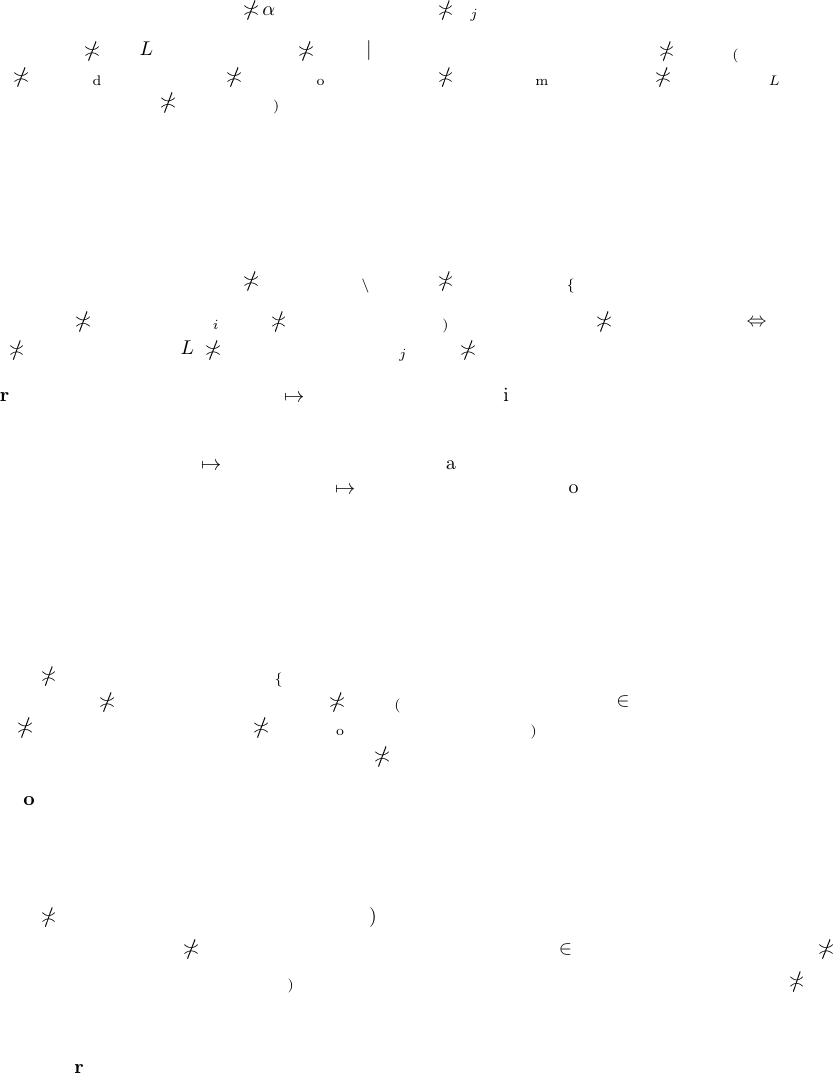Remark 82. To describe this, the deﬁnition of order poset is u sed twice. Let f and g are posets
of the same f orm A
hf i hgi i dom A: hf i
i
hgi
i
and hf i
i
hgi
i
L
Y
A|
(dom A)\{i}
: hf i
i
L hgi
i
L.
Theorem 83. f
pFCD(A)
g = f g for every pre-multifuncoids f and g of the same form A of
distributive lattices .
Proof. α
i
x =
def
f
i
x g
i
x. It is enough to prove that α is a multifuncoid.
We need to prove:
L
i
α
i
L|
(dom L)\{i}
L
j
α
j
L|
(dom L)\{j }
.
Really, L
i
α
i
L|
(dom L)\{i}
L
i
f
i
L|
(dom L)\{i}
g
i
L|
(dom L)\{i}
L
i
f
i
L|
(dom L)\{i}
L
i
g
i
L|
(dom L)\{i}
L
j
f
j
L|
(dom L)\{j }
L
j
g
j
L|
(dom L)\{j }
L
j
f
j
L|
(dom L)\{j }
g
j
L|
(dom L)\{j}
L
j
α
j
L|
(dom L)\{j }
.
Theorem 84.
F
pFCD(A)
F =
F
F for every set F of pre-multifuncoids of the same f orm A of join
inﬁnite distributive complete lattices.
Proof. α
i
x =
def
F
f F
f
i
x. It is enough to prove that α is a multifuncoid.
We need to prove:
L
i
α
i
L|
(dom L)\{i}
L
j
α
j
L|
(dom L)\{j }
.
Really, L
i
α
i
L|
(dom L)\{i}
L
i
F
f F
f
i
L|
(dom L)\{i}
⇔∃f F : L
i
f
i
L|
(dom L)\{i}
⇔∃f F :
L
j
f
j
L|
(dom L)\{j}
L
j
F
f F
f
j
L|
(dom L)\{j }
L
j
α
j
L|
(dom L)\{j}
.
Proposition 85. The mapping f
[f ] is an order embedding, f or multifuncoids of the form A of
separable starrish posets.
Proof. The mapping f
[f] is deﬁned because A are starrish poset. The mapping is injective
because A are separable posets. That f
[f ] is a monot one function is obvious.
Remark 86. This order embedding is useful to describe propert ie s of posets of pre-staroids.
Theorem 87. If f, g are multifuncoids of the same form A of distributive la ttices, then
f
pFCD(A)
g FCD(A).
Proof. Let A
f
pFCD(A)
g
and B A. Then for every k dom A
A
k
f
pFCD(A)
g
A|
(dom A)\{k}
=(f g)A|
(dom A)\{k}
=f (A|
(dom A)\{k}
) g(A|
(dom A)\{k}
).
Thus A
k
f(A|
(dom A)\{k }
) A
k
g(A|
(dom A)\{k}
); A [f ]A [g]; B [f ]B [g];
B
k
f(B |
(dom A)\{k}
) B
k
g(B |
(dom A)\{k}
); f(B |
(dom A)\{k }
) g(B |
(dom A)\{k}
) = (f
g)B |
(dom A)\{k}
=
f
pFCD(A)
g
B|
(dom A)\{k}
B
k
. Thus B
f
pFCD(A)
g
.
Theorem 88. If F is a set multifuncoids of the same form A of join iniﬁ nite distributive c omplete
lattices, then
F
pFCD(A)
f FCD(A).
Proof. Let A
h
F
pFCD(A)
f
i
and B A. Then for every k dom A.
A
k
F
pFCD(A)
F
A|
(dom A)\{k }
=(
F
F )A|
(dom A)\{k }
=
F
f F
f(A|
(dom A)\{k}
).
Thus f F : A
k
f(A|
(dom A)\{k}
); f F : A [f ]; B [f ]B [g]; f F : B
k
f(B |
(dom A)\{k}
);
F
f F
f(B|
(dom A)\{k }
) = (f g)B |
(dom A)\{k }
=
F
pFCD(A)
F
B|
(dom A)\{k}
B
k
.
Thus B
h
F
pFCD(A)
F
i
.
Conjecture 89. The formula f
FCD(A)
g cFCD(A) is not true in general for completary
multifuncoids (even for multifuncoids on powersets) f and g of the same form A.
Join of multifuncoids 11